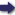(London :  Kegan Paul, Trench, Trübner & Co.,  1910.)

 Tools

## Search this bookPrev Page 27 Next``` ( 27 ) CHAPTER LII. ON THE CALCULATION OF ''AHAEGANA" IN GENEEAL, THAT IS, THE RESOLUTION OF YEAES AND MONTHS INTO DAYS, AND, VICE VEESi, THE COMPOSITION OF YEAES AND MONTHS OUT OF DAYS. The general method of resolution is as follows:—The General rule 1 • T -i 1 V J1 ^ ^°^ ^^ ^^^ complete years are multiplied by I2 ; to the product are th&sdvami-. added the months which have elapsed of the current ' '" year, [and this sum is multiplied by 30 ;] to this product are added the days which have elapsed of the current month. The sum represents the saurdhargana, i.e. the sum of the partial solar days. You write down the number in two places. In the one place you multiply it by S'^ii, i.e. the number which represents the universal adhimdsa months. The product you divide by 172,800, i.e. the number which represents the universal solar months. The quotient you get, as far as it contains complete days, is added to the number in the second place, and the sum represents the cand^rdhargana, i.e. the sum of the partial lunar days. The latter number is again written down in two different places. In the one place you multiply it by 55,739, i.e. the number which represents the universal unardtra days, and divide the product by 3,562,220, i.e. the number which represents the universal lunar days. The quotient you get, as far as it represents complete days, is subtracted from the number written in the second place, and the remainder is the sdvandhargana, i.e. the sum of civil days which we wanted to find. ```Prev Page 27 Next